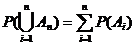# Statistics Assignment Help With Probability Function

## 2.6.1 Probability Function:

P(A) is the probability function defined on a σ – field B of events if the following properties hold:

1. For each A Є B, P(A) is defined, is real and P(A) ≥0
2. P(S) =1
3. {`If {An} is any finite or infinite sequence of disjoint events in B, then`}The above three axioms are termed as the axiom of positiveness, certainty and union.